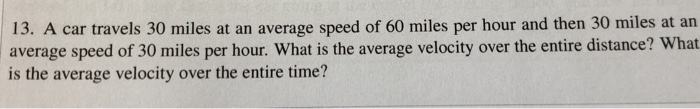# If 60 miles per hour is equivalent to a speed of 88 feet per second, express a speed of 45 miles per hour in feet per second

If 60 miles per hour is equivalent to a speed of 88 feet per second, express a speed of 45 miles per hour in feet per second.

feet/sec

66 ft/sec
##### Add Answer of: If 60 miles per hour is equivalent to a speed of 88 feet per second, express a speed of 45 miles per hour in feet per second
Similar Homework Help Questions
• ### convert 60 miles per hour to feet per second

convert 60 miles per hour to feet per second. (Hint: there are 5280 feet in a mile.

• ### 65 miles per hour is equal to how many feet per second

65 miles per hour is equal to how many feet per second?

• ### HOW LONG WILL IT TAKE A CAR BEING DRIVEN AT A SPEED OF 15 MILES PER HOUR TO TRAVEL 26,400 FEET

HOW LONG WILL IT TAKE A CAR BEING DRIVEN AT A SPEED OF 15 MILES PER HOUR TO TRAVEL 26,400 FEET? AN OBJECT MOVING AT A RATE 0F 15 MILES PER HOUR TRAVELS 22 FEET IN ONE SECOND.

• ### andys average driving speed for a 4-hour trip was 45 miles per hour

andys average driving speed for a 4-hour trip was 45 miles per hour. during the first 3 hours he drove 40 miles per hour. what was his average speed for the last hour of his trip? The trip length was 4 x 45 = 180 miles. He went 3x40 = 120 the first three hours. How many miles does that leave for the last hour?4+5

• ### If the wind is blowing at 54 feet per second, how many miles per hour is it

If the wind is blowing at 54 feet per second, how many miles per hour is it?

• ### Chan drove for X miles at 45 miles an hour, Y miles at 60 miles an hour, and Z miles at 55 miles an hour

Chan drove for X miles at 45 miles an hour, Y miles at 60 miles an hour, and Z miles at 55 miles an hour. Which expression can be used to find the total numbers of hours that Chan drove?A. (45+60+55)(XYZ)B. 45X + 60Y + 55ZC. (45+60+55)/XYZD. X/45 + Y/60 + Z/55

• ### Ryan is batting a baseball, he presently can cover the 90 foot distance with the average speed of 20 feet per second What is the average speed in miles per hour

Ryan is batting a baseball, he presently can cover the 90 foot distance with the average speed of 20 feet per secondWhat is the average speed in miles per hour?

• ### 13. A car travels 30 miles at an average speed of 60 miles per hour and...13. A car travels 30 miles at an average speed of 60 miles per hour and then 30 miles at an average speed of 30 miles per hour. What is the average velocity over the entire distance? What is the average velocity over the entire time?

• ### Jimmy is traveling at a speed of 135 miles per hour (mph)

Jimmy is traveling at a speed of 135 miles per hour (mph). What is his speed in feet per second? Include units in the calculation to show the unit conversion.

Free Homework App